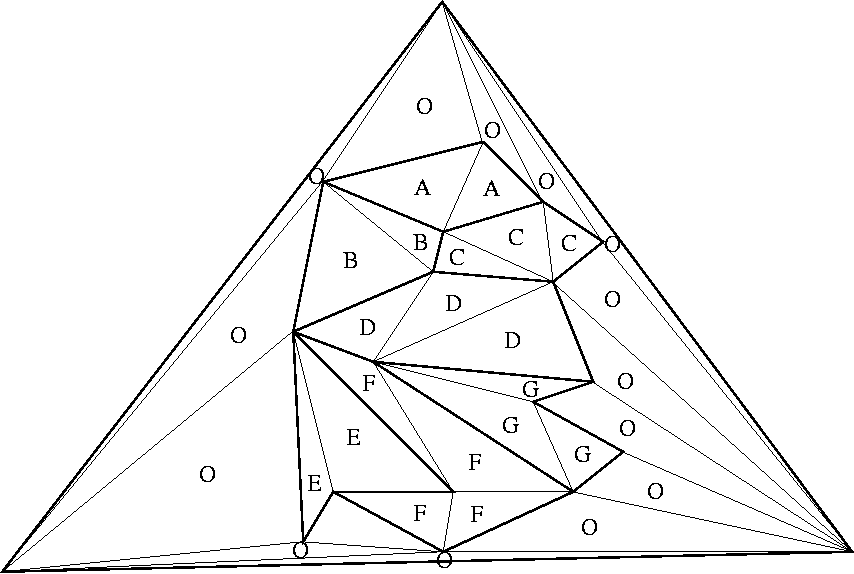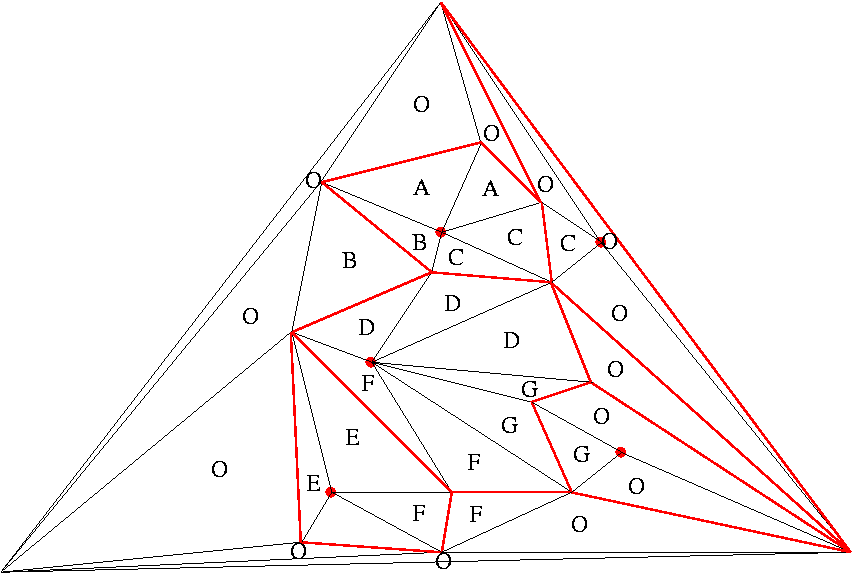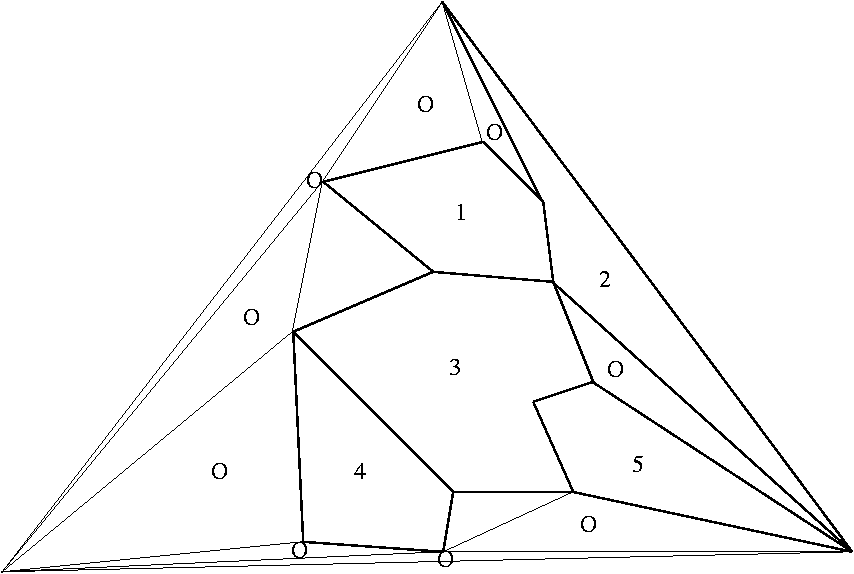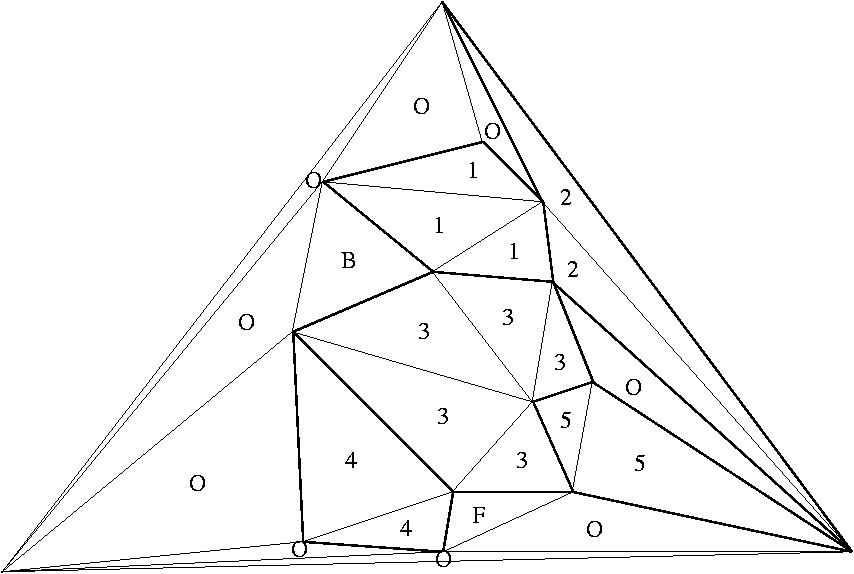Previous Demonstration | About Next

Constructing the Data Structure:

First of all, to make things simpler, we enclose the whole triangulation in a large enough triangle in such a way that none of its edges intersect the others. This will represent the outter face (labeled here as "O"):We take the obtained graph and we process it in the following way:
1. We find an independent set of vertices with degree less than or equal to 8 inside the hull:2. We remove them from the graph, obtaining independent holes:3. We retriangulate these holes (by following the same rule for labels as before):4. We repeat the above steps until we are left with 3 vertices (the large triangle).

 Previous Demonstration | About Next